# Mathematical problems for self assessment

###### APPLICATIONS OF INTEGRATION I

For Questions 1 and 2 you can assume the following formulae: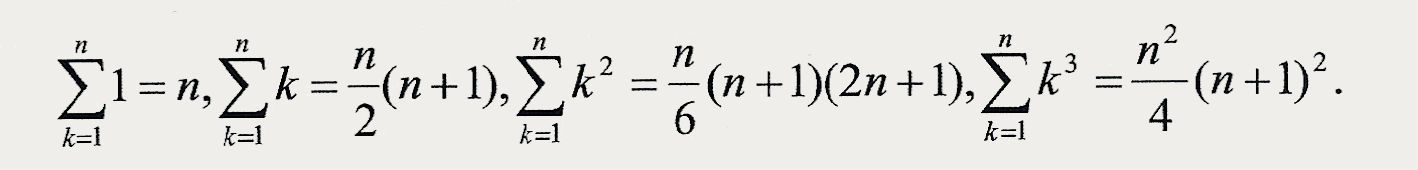1. Find the area under the curvefrom x = 0 to x = 1 using the limit of a sum technique.

[Ans.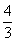].

2. Find the area under the curvebetween x = 0 and x = 2 using the limit of a sum technique.

[Ans. 8].

3. Find the volume of the solid formed when the functionbetweenand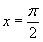is rotated about the x axis.

[Ans.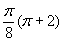].

4. Find the volume of the solid formed when the cusp function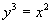is rotated about the y axis betweenand.

[Ans.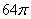].

5. Find the co-ordinates of the centre of mass of the plane lamina given in the figure below:[Ans.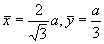].

6. Find the co-ordinates of the centre of mass of the plane lamina given in the figure below:[Ans.].

7. Find the co-ordinates of the centre of mass of the plane lamina given in the figure below: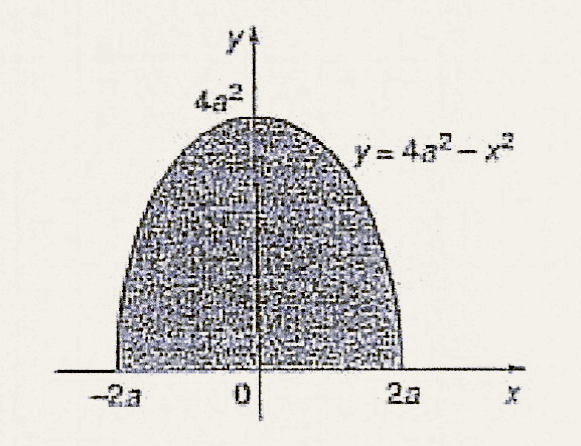[Ans.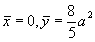].